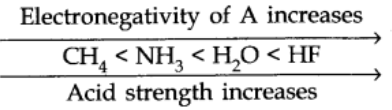# Arrhenius Concept of Acids and Bases

## Arrhenius Acids:

According to Arrhenius theory, acids are substances that dissociates in water to give hydrogen ions H+(aq).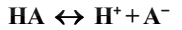## Arrhenius Bases:

Bases are substances that produce OH(aq) after dissociation in water.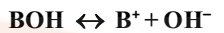### Limitations of the Arrhenius Concept

• According to the Arrhenius concept, an acid gives H+ ions in water but the H+ ions does not exist independently because of its very small size (~H-18 m radius) and intense electric field.
• It does not account for the basicity of substances like, ammonia which does not possess a hydroxyl group.

# Bronsted-Lowry Acids and Bases

## Bronsted-Lowry Acids:

Those compounds which can transfer protons that is H+ to other compounds.

## Bronsted-Lowry Bases:

Those compounds which can accept protons.

That is, Bronsted acids are “proton donors” and Bronsted bases are “Proton acceptors”.# Acid and Base as Conjugate Pairs

The acid-base pair that differs only by one proton is called a conjugate acid-base pair.
Let us consider the example of ionization of HCl in water.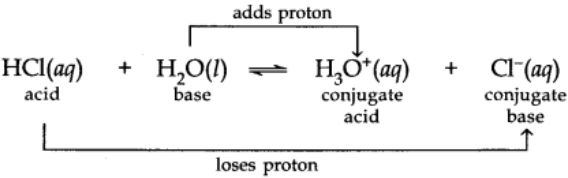Here water acts as a base because it accepts the proton.
CL is a conjugate base of HCl and HCl is the conjugate acid of base CL. Similarly, H20 is conjugate base of an acid H30+ and H30+ is a conjugate acid of base H2O.

# Lewis Concept

## Lewis Acid:

A compound which can accept a pair of electrons.

## Lewis Base:

is a compound which can transfer its lone pair of electrons.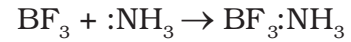In the above reaction BF3 is a Lewis acid and NH3 is a Lewis base.

## Calculation of the Degree of Dissociation (α) from Density Measurement

α= (D-d)/d

Where, D = theoretical vapour density

d = observed vapour density

Now, molecular mass = 2 * VD

α= (MC– M0)/M0

where, MC = calculated molecular weight

M0 = observed molecular weight

# Ionization of Acids and Bases

Strength of acid or base is determined with the help of extent of ionization in aqueous solution.

# pH Scale:

Hydrogen-ion concentration are measured as the number of gram ions of hydrogen ions present per liter of solution. Since these concentrations are usually small, the concentration is generally expressed as the pH of the solution. pH being the logarithm of the reciprocal of the hydrogen ion concentration.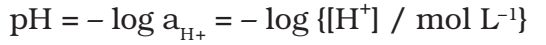pH of pure water at 250C is given as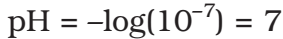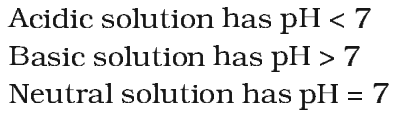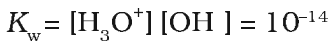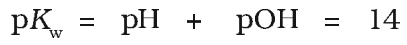# Di and Polybasic Acids

Acids which contain more than one ionizable proton per molecule are called Dibasic acids or polybasic acids or polyprotic acids.
Common examples are oxalic acid, sulphuric acid, phosphoric acid etc.

The ionization reactions for a dibasic acid can be represented as: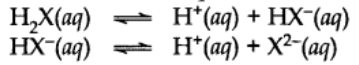Their equilibrium constants can be written as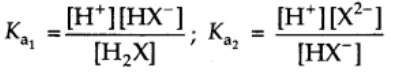Ka1 and Ka2 are called first and second ionization constants respectively.

For dibasic acid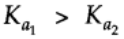## Factors Affecting Acid Strength

When the strength of H-A bond decreases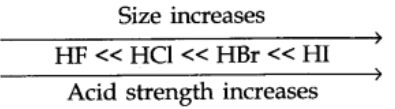The energy required to break the bond decreases, H-A becomes a stronger acid.
As the size of A increases down the group, H-A bond strength decreases and so the acid strength increases.
In a period, as the electronegativity of A increases, the strength of the acid increases.